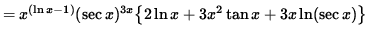SOLUTIONS TO LOGARITHMIC DIFFERENTIATION

SOLUTION 1 : Because a variable is raised to a variable power in this function, the ordinary rules of differentiation DO NOT APPLY ! The function must first be revised before a derivative can be taken. Begin with

y = xx .

Apply the natural logarithm to both sides of this equation getting.

Differentiate both sides of this equation. The left-hand side requires the chain rule since y represents a function of x . Use the product rule on the right-hand side. Thus, beginning with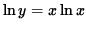and differentiating, we get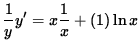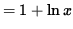.

Multiply both sides of this equation by y, getting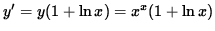.

SOLUTION 2 : Because a variable is raised to a variable power in this function, the ordinary rules of differentiation DO NOT APPLY ! The function must first be revised before a derivative can be taken. Begin with

y = x(ex) .

Apply the natural logarithm to both sides of this equation getting.

Differentiate both sides of this equation. The left-hand side requires the chain rule since y represents a function of x . Use the product rule on the right-hand side. Thus, beginning withand differentiating, we get(Get a common denominator and combine fractions on the right-hand side.)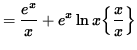(Factor out ex in the numerator.)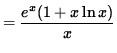.

Multiply both sides of this equation by y, getting(Combine the powers of x .)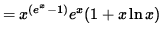.

SOLUTION 3 : Because a variable is raised to a variable power in this function, the ordinary rules of differentiation DO NOT APPLY ! The function must first be revised before a derivative can be taken. Begin with

y = (3x2+5)1/x .

Apply the natural logarithm to both sides of this equation getting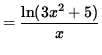.

Differentiate both sides of this equation. The left-hand side requires the chain rule since y represents a function of x . Use the quotient rule and the chain rule on the right-hand side. Thus, beginning withand differentiating, we get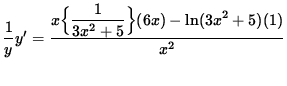(Get a common denominator and combine fractions in the numerator.)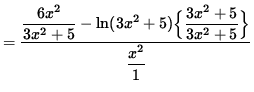(Dividing by a fraction is the same as multiplying by its reciprocal.)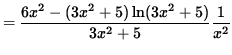.

Multiply both sides of this equation by y, getting(Combine the powers of (3x2+5) .).

SOLUTION 4 : Because a variable is raised to a variable power in this function, the ordinary rules of differentiation DO NOT APPLY ! The function must first be revised before a derivative can be taken. Begin with.

Apply the natural logarithm to both sides of this equation getting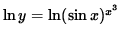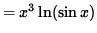.

Differentiate both sides of this equation. The left-hand side requires the chain rule since y represents a function of x . Use the product rule and the chain rule on the right-hand side. Thus, beginning with truein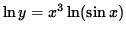and differentiating, we get(Get a common denominator and combine fractions on the right-hand side.).

Multiply both sides of this equation by y, getting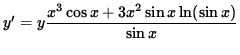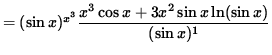(Combine the powers of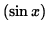.).

SOLUTION 5 : Because a variable is raised to a variable power in this function, the ordinary rules of differentiation DO NOT APPLY ! The function must first be revised before a derivative can be taken. Begin with.

Apply the natural logarithm to both sides of this equation and use the algebraic properties of logarithms, getting.

Differentiate both sides of this equation. The left-hand side requires the chain rule since y represents a function of x . Use the product rule and the chain rule on the right-hand side. Thus, beginning withand differentiating, we get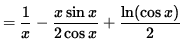(Get a common denominator and combine fractions on the right-hand side.)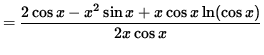.

Multiply both sides of this equation by y, getting(Divide out a factor of x .)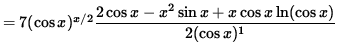(Combine the powers of.).

SOLUTION 6 : Because a variable is raised to a variable power in this function, the ordinary rules of differentiation DO NOT APPLY ! The function must first be revised before a derivative can be taken. Begin with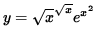.

Apply the natural logarithm to both sides of this equation and use the algebraic properties of logarithms, getting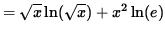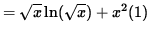.

Differentiate both sides of this equation. The left-hand side requires the chain rule since y represents a function of x . Use the product rule and the chain rule on the right-hand side. Thus, beginning withand differentiating, we get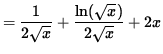(Get a common denominator and combine fractions on the right-hand side.)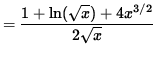.

Multiply both sides of this equation by y, getting(Combine the powers of.).

SOLUTION 7 : Because a variable is raised to a variable power in this function, the ordinary rules of differentiation DO NOT APPLY ! The function must first be revised before a derivative can be taken. Begin with.

Apply the natural logarithm to both sides of this equation and use the algebraic properties of logarithms, getting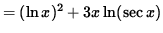.

Differentiate both sides of this equation. The left-hand side requires the chain rule since y represents a function of x . Use the product rule and the chain rule on the right-hand side. Thus, beginning withand differentiating, we get(Divide out a factor of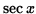.)(Get a common denominator and combine fractions on the right-hand side.).

Multiply both sides of this equation by y, getting(Combine the powers of x .)